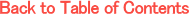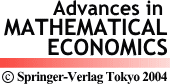Adv. Math. Econ. Volume 6, pp.55-68 (2004)

Recursive methods in probability control

Seiichi Iwamoto
Department of Economic Engineering, Graduate School of Economics, Kyushu University 27, Hakozaki 6-19-1, Higashiku, Fukuoka 812-8581, Japan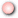PDF styleDVI style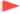In this paper we propose recursive methods in probability control. We maximize the threshold probability that a ``total" reward is greater than or equal to a given lower level. We take three types of reward function --- general function, forward recursive function and backward recursive function. By three types of imbedding method, we derive a backward recursive equation for maximum probability function. The first is to view any history (alternating sequence of state and decision) as a new state. The second is to incorporate past values (cumulative rewards) in state dynamics. The third is a state expansion by attachment of future values (threshold levels). It is shown that the threshold probability problem has an optimal policy in a common large class.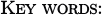recursive function, probability control, dynamic programming, invariant imbedding, forward recursive, backward recursive, backward recursive formula, forward recursive formula, past values, cumulative rewards, future values, threshold levels, expanded Markov policy, general policy, primitive policy